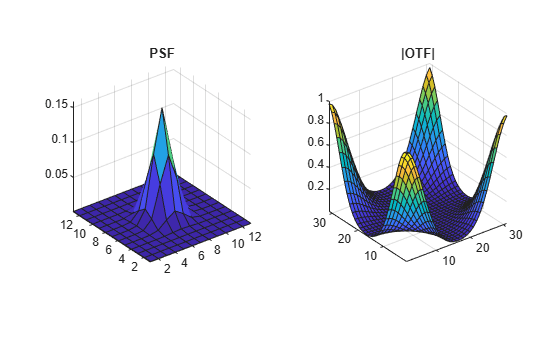psf2otf

Convert point-spread function to optical transfer function

Description

example

OTF = psf2otf(PSF) computes the fast Fourier transform (FFT) of the point-spread function (PSF) array and creates the optical transfer function array, OTF, that is not influenced by the PSF off-centering.

OTF = psf2otf(PSF,sz) specifies the size, sz, of the optical transfer function.

Examples

collapse all

PSF  = fspecial('gaussian',13,1);

Convert the PSF to an Optical Transfer Function (OTF).

OTF  = psf2otf(PSF,[31 31]);

Plot the PSF and the OTF.

subplot(1,2,1);
surf(PSF);
title('PSF');
axis square;
axis tight
subplot(1,2,2);
surf(abs(OTF));
title('Corresponding |OTF|');
axis square;
axis tightInput Arguments

collapse all

Point-spread function, specified as a numeric array of any dimension.

Example: PSF = fspecial('gaussian',13,1);

Data Types: single | double | int8 | int16 | int32 | int64 | uint8 | uint16 | uint32 | uint64
Complex Number Support: Yes

Size of the output optical transfer function OTF, specified as a vector of positive integers. The size of OTF must not exceed the size of PSF in any dimension. By default, OTF is the same size as PSF.

Data Types: double

Output Arguments

collapse all

Optical transfer function, returned as a numeric array of size sz.

Data Types: double
Complex Number Support: Yes

Tips

• To ensure that OTF is not altered because of PSF off-centering, psf2otf postpads PSF (down or to the right) with 0s to match dimensions specified in sz. Then psf2otf circularly shifts the values of PSF up (or to the left) until the central pixel reaches (1,1) position.

• This function is used in image convolution and deconvolution when the operations involve the FFT.